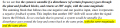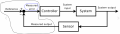# Stability bode diagram Phase margin and gain margin

#### leo0001

Joined Sep 30, 2018
79
Hi,I understand this assertion. But i'm actually not understand why a system would be stable if a disturbance goes around the loop and come back to the same point, with a higher magnitude, and a different phase ? If the gain is inferior to 1, whatever is the phase, i can understand that the disturbance will diseaper after passing several times in the loop... Please tell me where is my error(I know from the transfer function that it would be unstable if GH is equal to -1, which correspond to a gain = 0 dB and a phase equal to -180 °)

Suppose the gain GH is higher than 1 and equal to G and the phase shift is equal to P and is not equal to -180 ° at the frequency f. I have a disturbance which occurs after the summing node. The disturbance is equal to Asin(wt) with w = 2pi*f. At the same point (after the summing node), the disturbance would be equal to G*Asin(wt + P -180). So now we have a disturbance which is higher than the previous... and it is just shifted in phase from the previous...

Thank you very muchLast edited:

#### crutschow

Joined Mar 14, 2008
32,032
It's the frequency at which the feedback returns in-phase that's of most concern.
If the gain is near one or higher at that frequency then there is an instability.
That's near or equal to the frequency which shows as ringing or oscillation in the system response.
The phase-shift and gain around the loop change with frequency, so that's why you use both gain and phase-margin to determine the loop stability.
Make sense?

•leo0001

#### leo0001

Joined Sep 30, 2018
79
Thank for your reply. Suppose I have a disturbance at the frequency w (radian/seconds). The phase of the disturbance returns in phase in the loop at the same point where the disturbance was coming, ie, phase(G(s)*H(s)) = -180 ° or 180 ° where s = jw. The closed loop gain is G/(1+GH). If the gain of the open loop is equal to 1 and the phase is 180 or -180°. The close loop gain will be equal to infinity, nevertheless if the gain of the open loop is higher than 1, the close loop gain will not be equal to infinity. And if I suppose the gain of the open loop equal to 100, whatever the phase, the closed loop gain would not be unstable. Did i do a mistake ?

#### Jony130

Joined Feb 17, 2009
5,445
•leo0001

#### leo0001

Joined Sep 30, 2018
79
Hi,

Thank you for your links ! I found some informations which are really interesting ! (notably the link between phase margin and the response time (gain) according to bode diagramm analysis). I saw your commentsYou are really active !

Nevertheless, I still do not get the principle ... I actually don't understand why the phase is important.

Here we go:

#### crutschow

Joined Mar 14, 2008
32,032
I actually don't understand why the phase is important
Why would it not be important?
It's the in-phase component that generates any possible instability.
If you can't understand that, then I suggest you just accept it, since I don't know how to further explain it beyond the articles you've read.#### leo0001

Joined Sep 30, 2018
79
Hello,

Thank you for your answer. In the previous articles that Jony sent to me and I thank him for thoses articles, I find no comment about why it is stable with a gain higher than 1 whatever the phase.

So you can explain if you know the answerThank you,

#### Jony130

Joined Feb 17, 2009
5,445
I find no comment about why it is stable with a gain higher than 1 whatever the phase.
Who told you that the circuit will be stable? If the phase condition is met (positive feedback ) and the loop gain is Aol*β > 1. The circuit will oscillate.

#### leo0001

Joined Sep 30, 2018
79
Hi,

What do you by "If the phase condition is met" ? if the phase shift of the open loop transfer function is equal to 180 ° or -180 ° ?What is actually a positive feedback ? By positive feedback I understand that the reference minus the output returned by the feedback gives a result higher than the reference . On an AOP, it means that Vnon inverting input minus Vinverting input is higher than the V non inverting input. (V+ - V- > V+).

Thank you,